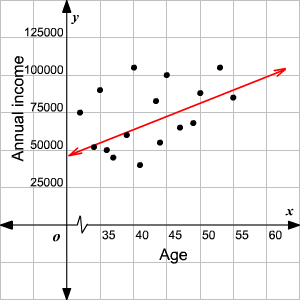# Line of Best Fit(Eyeball Method)

A line of best fit is a straight line drawn through the maximum number of points on a scatter plot balancing about an equal number of points above and below the line.

It is used to study the nature of relation between two variables.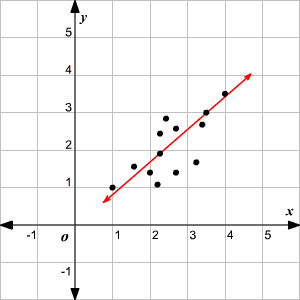The line of best fit in the scatter plot above rises from left to right; so, the variables have a positive correlation .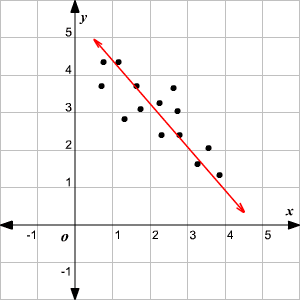Here, the line of best fit drops from left to right, so the variables have a negative correlation.

Example 1:

Draw a line of best fit for the scatter plot given.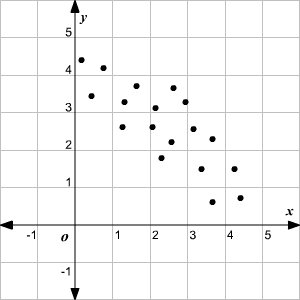Solution:

Draw a line through the maximum number of points, balancing about an equal number of points above and below the line.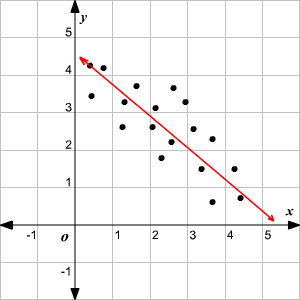Example 2:

Draw a line of best fit for the scatter plot given.

 Age of a Person (in years) ( $x$ ) Annual Income (in \$)( $y$ ) $32$ $75000$ $40$ $110000$ $35$ $90000$ $36$ $50000$ $37$ $45000$ $39$ $60000$ $34$ $51000$ $39$ $60000$ $41$ $40000$ $45$ $100000$ $47$ $65000$ $49$ $68000$ $53$ $105000$ $55$ $85000$ $43$ $80000$ $44$ $55000$ $50$ $85000$

Solution:

Plot the age in the $x$ -axis and the income in the $y$ -axis and mark the points.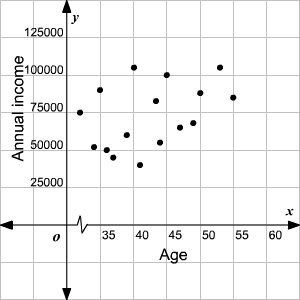Draw a line through the maximum number of points balancing about an equal number of points above and below the line.